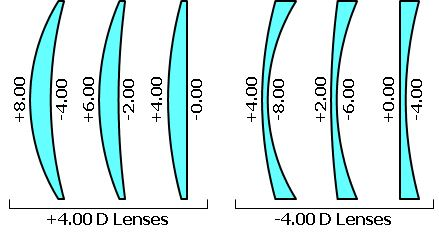Physics

# How to Measure Power of a Lens

Power of a lens is a measure of the degree of convergence or divergence of light falling on it. The power of a lens (P) is defined as the reciprocal of its focal length.

P = 1/fThe unit of power is dioptre (D): 1 D = 1 m-1. The power of the lens is said to be 1 dioptre if the focal length of the lens is 1 metre. P is positive for converging lens and negative for diverging lens. Thus, when an optician prescribes a corrective lens of power + 0.5 D. the required lens is a convex lens of focal length + 2 m. A power of -2.0 D means a concave lens of focal length -0.5 m.